SELF 2 S.B. Karavashkin and O.N. Karavashkina2. Citation: ”I have already answered the question of Jacobian equal to unity, while the postulates of GR still have to be proven.” Not only in GR but in classical Newtonian mechanics we can do arbitrary coordinate transformations, if we fulfil two conditions: a) the Jacobian of transformation has to be non-zero (it to have the reverse transformation), b) at the same time we have to make corresponding changes in the equation of motion. Solve any problem with any coordinate transformation (fulfilling these conditions), then you can pass to the initial coordinates - your answer will be correct. Schwarzschild has fulfilled the item ‘a)’, using the composition of two transformations with Jacobian equal to unity (i.e. non-zero). He had not to fulfil the item ‘b)’ by a simple reason: field equations are not changed in coordinate transformation with Jacobian equal to unity. What is the problem? In this your question we find several topics which we considered before. First of all, when saying, “Not only in GR but in classical Newtonian mechanics we can do arbitrary coordinate transformations, if we fulfil two conditions…”, you are meaning not simply a free transition from one reference frame (RF) to another but that the principle of physical laws equivalence in inertial and non-inertial RFs is legal. But the equivalence principle and possibility to freely pass from one RF to another are different things. If in classical physics we can pass from a non-inertial RF (NRF) to that inertial (IRF), we have certainly to account that it is non-inertial, which will basically change the laws of interaction in this RF. In particular, in passing usually appear fictitious forces of inertia, the body’s trajectory considerably changes. Even vectorial interrelations can change, nothing to say of conservation laws. Again, if speaking of equivalence in relativistic meaning, the inertial force and gravity force affect the body in the same way, while in classical mechanics they affect differently. If we recall Einsteinian sealed carriage accelerated by forces unknown for the observer, an educated observer will immediately distinct the affection of one force from another. Actually, if the locomotive accelerates, the inertia force will press the observer into the chair, as this force is contacting and affects the coupling of locomotive and carriage, then on the shell of carriage, and the carriage, in its turn, affects the chair, and only the chair affects the observer’s ‘gentle place’.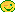In other words, inertial forces always mean the affection in a chain, from body to body. This causes the vibrations that are used to start the motion of heavy trains. Because of it we feel the affection of inertial force. As opposite to this, gravity forces affect all bodies in the field simultaneously, or rather independently on each body. Namely because of it all bodies in a gravity field fall with the same acceleration independent of mass. And namely so the observer freely falling in a gravity field does not feel the force affection. Only if the observer had the size comparable with those at which the strength of gravity field considerably changes, he would be able to feel the strength of this field affection. In limits of carriage it is senseless to speak of it.Thereupon, applying this understanding to our observer, we can conclude that in turning on some virtual gravity field, the observer will accelerate just as the carriage; this means, he will not feel this field, although in relation to the inertial external observer he will move with an acceleration. Thus, the physical laws in inertial and non-inertial RFs are inequivalent, and the very equivalence principle is wrong. While you are reducing this all to Jacobian and related changes of motion equations. The whole question is, to what the equations of motion have to correspond? To the warping of space? Physics is not geometry, or people would not need to introduce a new name of science different from geometry. Here takes place a standard mistake of many colleagues for whom IRF is habitual as slippers; often they even do not note it when using. To understand it, suppose, we are located in some RF and have to reveal the regularities of motion of trial body. We will first throw the body in different directions and yield different results, though the catapult is same. Strange? Our experience shows so. Then we will try to clear, how this body bounces back from a hindrance - and see, it bounces to a larger distance than that from which we threw it. If we change the hindrance’s orientation, the bouncing coefficient also changes. Finally, we will decide to determine, how the body moves when it is pushed strongly in one direction - and see, dependently on direction the trajectory can be direct either curvilinear. Then, to generalise our experience, we willy-nilly begin to sum something up either to subtract, to find the IRF in which all these motions would have their common. This is objectively, since in a NRF we are helpless. If the conditions of non-inertiality change, the laws of interaction essentially change. And only with respect to IRF we are able to determine the type of these transformations. We can recall as an example different shape of riverbanks. Until the Earth was thought to be the centre of the universe and all stars, planets and the Sun orbited around it, this long-time-known regularity could not be explained. This reveals wrong position of Mach who tried to identify our own motion with our motion with respect to the universe. Only when the Earth’s orbiting was established and there appeared the basis to model IRF with reference to which the Earth is orbiting, the effect was explained. Today we rarely mention this IRF but, simplifying, speak of Coriolis forces. But if we need to model the problem with full accuracy, it will be necessary to introduce this IRF. To generalise, we can say, in solving of any nonlinear problems there in the model always is present the IRF, though often our simplifications hide it. All regularities in their general appearance which we can use in our analysis are true only in IRF. In a NRF we can yield only some particular regularities inherent in this specific trajectory of body’s motion, and we may not generalise these regularities within the NRF without passing to IRF. The second part of your question is, basically, not so much related to the first. Actually, to yield associative result, we have to return after all calculations to the initial coordinates which we have put into the model. We indirectly pointed you it in the post and then, seeing that you did not understand stated it in the first supplement. Of course, we are pleased that you understood this nuance and took it so naturally that now you are trying to explain it for us.Now it remains you to understand, it was one of basic Schwarzschild’s mistakes of which we wrote in our first supplement, answering your question, what is Schwarzschild’s mistake. In the light of the said, your emphasis “that the field equations do not change in coordinate transformation with Jacobian equal to the unity” is irrelevant to the requirement to pass to the initial coordinates in order to make the solution associative to the statement of problem. We showed it in the example with the metric of Einstein who, having passed to one more RF, escaped the event horizon and singularity in the centre. Was he right? Undoubtedly, no, we can easily show it without any relativistic metrics. See, let us take Newton’s gravity law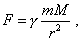(11)
 introduce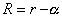(12)
 and yield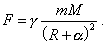(13)
 You are thinking, we have a right to conclude from (13) that in Newton’s law the singularity is absent. Is it so?No of course. In changing the variable, we above (12) introduce also the domains of its variation. If in our example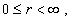(14)
 then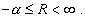(15)
 It follows from (15) that in (13) the singularity will be present but at other values of used variable R . And nothing of surprise that the variable R can take negative values. This is not the measured variable. The measured value is r and for it the requirement of non-negative radius-vector in spherical either polar coordinates is true. The same in Schwarzschild’s problem. Which relation has the Jacobian equal to unity to the return to initial variables? No relation. In the initial metric, there was the variable r. In the final expression Schwarzschild passed to the variable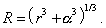(16)
 This means, as in spherical coordinates the radius-vector cannot be negative, there acts the condition similar to (14), and acts namely with respect to r. In accordance with this condition we yield for R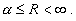(17)
 The singularity in the Schwarzschild’s final expression, at the lower limit of domain of r variation, will clearly reveal in new coordinates at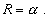(18)
 But this does not mean any event horizons out of the centre of gravitating body. The centre in the new coordinate system will be determined by the condition (18), and the domain of R variation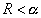(19)
 is discrepant to the physical stipulations of the model, as it contradicts the domain of existence of the very variable R. Your difficulty was in misunderstanding this.Contents: / 1 / 2 / 3 / 4 / 5 / 6 / Paper